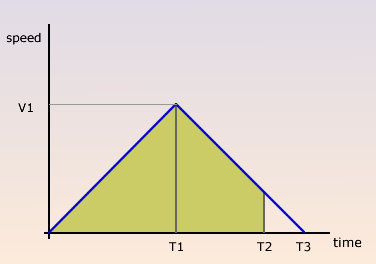Formula1 Rat Race – How fast is that?

By | November 25, 2007

The schools competition includes a head-to-head drag race called Formula 1. If you want to know how fast you have to be, read this.The Formula 1 Rat Race track is straight and 7.2m long. At the start end is an area 375mm long where the rat starts from. At the end is a 1.2m long area for the mouse to come to a rest. It must not overshoot this area of penalties will be incurred that will almost certainly eliminate the rat from the competition.For best performance, a rat will probably want to accelerate from the start line as hard as it can and then brake as hard as it can to bring it to a rest just short of the end of the braking area. This means a run of total length about 6.8m with the rat crossing the line as fast as it can while still allowing space to come to a halt.We can plot the mouse speed against time:At time T1 the mouse will be half way along its path having travelled 3.4m. The time taken to get there (T1) can be calculated from the standard equations of motion as:

T1 = sqrt(2*3.4/a)

where a is the acceleration of the rat. By inspection, it can be seen that T3 is twice T1.T2 is the time that the rat crosses the finishing line. Since we know the distance between the finishing line and the end of the braking area (1.2m), we can use the same equations to determine the time interval T3-T2 which is:

T3-T2 = sqrt(2*1.2/a)

Which is easily rearranged to give:

T2 = T3 – sqrt(2.4/a)

Substituting 2*T1 for T3 in this equation gives us:

T2 = 2*sqrt(6.8/a) – sqrt(2.4/a)

Simplifying:

T2 = 3.67/sqrt(a)

Now we can make a table to see how long the race will take for different values of acceleration, a:

 Acceleration (m/s/s) time (s) 1 3.66 2 2.59 3 2.11 4 1.83 5 1.63 6 1.49 7 1.38

I believe the current record holder from the 2007 competition managed to run in 2.58 seconds indicating the equivalent of continuous acceleration/deceleration of about 2m/s/s.A good micromouse can easily manage accelerations of better than 3m/s/s. Things start to get tricky at more than 5m/s/s.Thus, I think we should be expecting times for this competition to fall below 2 seconds and, for the ambitious, a target time of 1.5 seconds would be appropriate.So, give it a go.But hurry.

This site uses Akismet to reduce spam. Learn how your comment data is processed.